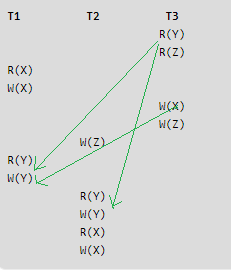Related Articles
ISRO | ISRO CS 2011 | Question 15
• Last Updated : 14 May, 2018

What is the equivalent serial schedule for the following transactions?

```T1          T2          T3
R(Y)
R(Z)
R(X)
W(X)
W(Y)
W(Z)
W(Z)
R(Y)
W(Y)
R(Y)
W(Y)
R(X)
W(X)                                ```

(A) T1 − T2 − T3
(B) T3 − T1 − T2
(C) T2 − T1 − T3
(D) T1 − T3 − T2

Answer: (B)

Explanation: From the following precedence graph, T3–>T1–>T2So, option (B) is correct.

Quiz of this Question

Attention reader! Don’t stop learning now. Get hold of all the important DSA concepts with the DSA Self Paced Course at a student-friendly price and become industry ready.

My Personal Notes arrow_drop_up